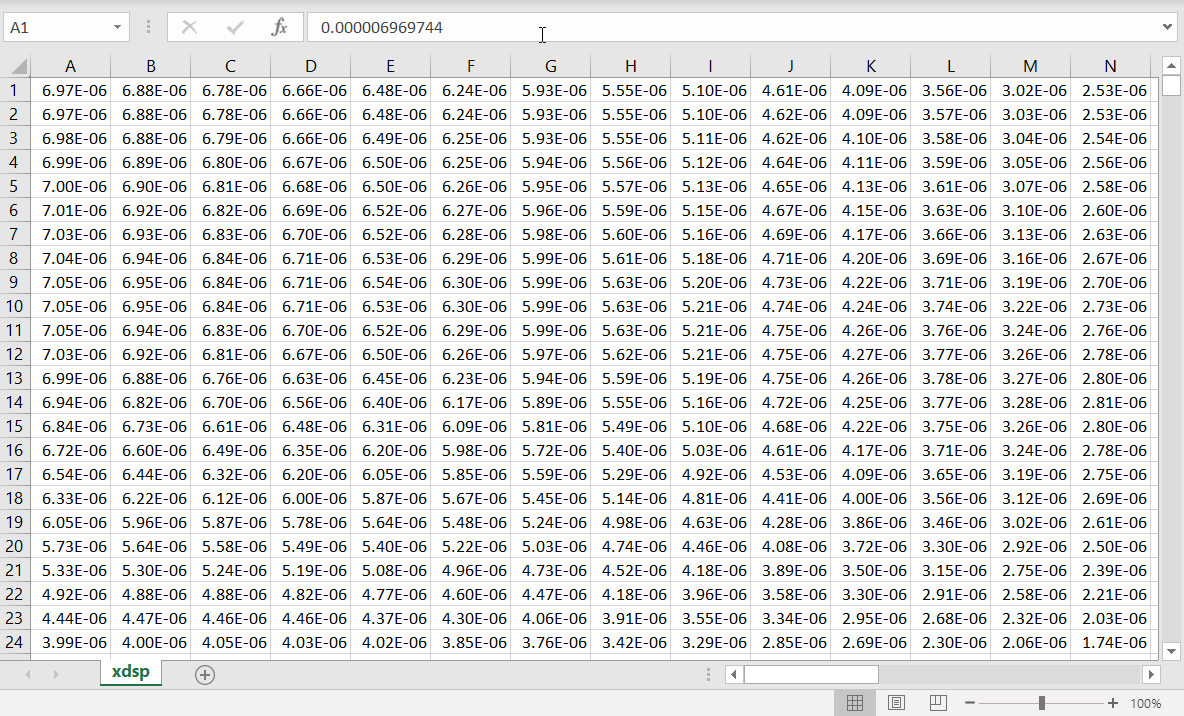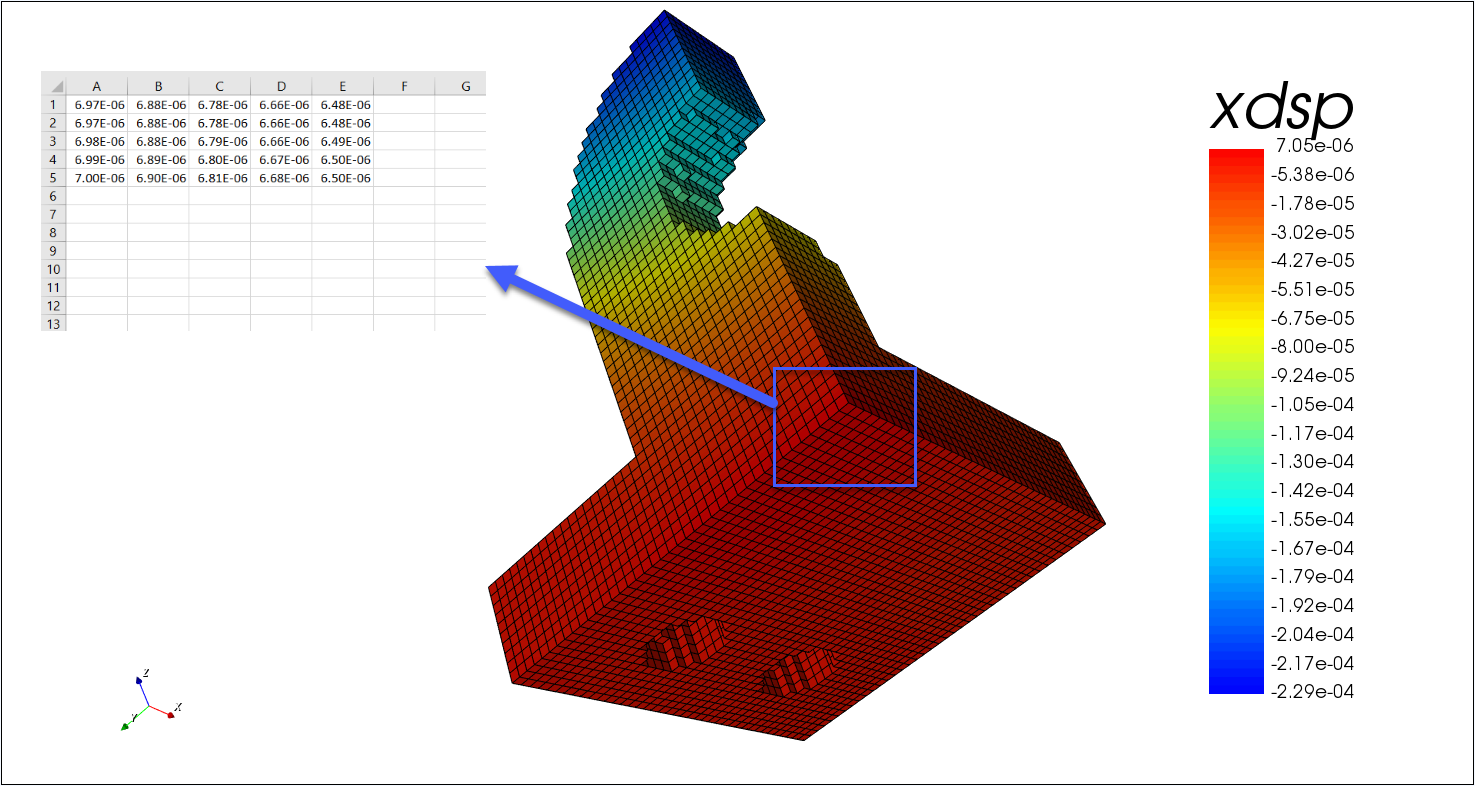# Exporting Array Data into Text or CSV

To export data arrays into text or csv format, the following code can be used:

```read d1 snapshot.flxdato

data cddo d1/1/xdsp 'xdsp.csv'
```

Note: This code suppose that the array "xdsp" corresponding to the mechanical displacement in the x direction has been calculated and exported in the snapshot.flxdato file

This will generate a file like this one:The CDDO command is used to write values contained within a Data Manager array to a disk file in a comma-delimited format that can be imported into EXCEL or some other program. This command is typically used to output a 2D section of a 2D or 3D array. It will also write out a 3D region if an array is requested.

The typical cddo syntax is:

```  data       cddo dataname filename ibegin iend jbegin jend kbegin kend
```

If the array is 3D, the specified indices should form a 2D section of the array if the data is to be imported into a program such as EXCEL.

If the data being written has a constant I-index, i.e., only the J- and K- indices vary, then each line of data written to the file has a constant K-index and the J-index varies along the line. For a constant J-index section, each line of data is for a constant K-index. For a constant K-index section, each row of data is for a constant J-index.

If a 3D array (or subregion of the array) is written to a file, each line of data has a constant J- and K-index, and the I-index varies along the line. The first line of data will be for J = jbegin, K = kbegin. The second line is for J = jbegin+1, K= kbegin, etc. I.e., the J-index permutates first and then the K-index.

Example:

```read d1 snapshot.flxdato

data cddo d1/1/xdsp 'xdsp.csv' 1 5 1 5 1 1
```

This code exports for example only the results at element center of the elements with 1<i<5 and 1<j<5 of the layer k = 1

This is what you would get:To export ALL the elements of one layer k = 1, you can use the following code:

```read d1 snapshot.flxdato

data cddo d1/1/xdsp 'xdsp.csv' * * * * 1 1
```

## How to export data at specific coordinates?

1- First, make sure that you have the "out modl" in your data output. This is what will include the model data into the result flxdato file (coordinates, nodes...)

```data
out modl
out shap/all
out pmax
end
```

2- Use the following review script

```/* Read data file

/* Specify xy position to get pressure
symb px = 2.e-3
symb py = 5.e-3

/* Plot max pressure
grph
plot d1/1/pmax
end

/* Get closest node to location
symb #get { ii ij } clsnode d1 \$px \$py

/* Get pressure
symb #get { pval } array d1/1/pmax \$ii \$ij

/* Report to user and write to file
symb #msg 1 &> 'pres.txt'
The pressure at x=\$px y=\$py is \$pval Pa

term
```

This script will find the closest nodes corresponding to the px,py position and output the pressure value at that position

Then it will write those variables into a text file.

Note: For some complex applications, it may be easier to export your data in MATLAB and post-process in matlab with custom scripts (You can also open matlab files with Python)